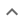•# Factoring multivariable polynomials

We think you wrote:

###This solution deals with factoring multivariable polynomials.

Solution found(2x+9y)^2

## Step by Step Solution## Step  1  :

#### Equation at the end of step  1  :

  ((4 • (x2)) +  36xy) +  34y2
Step  2  :

#### Equation at the end of step  2  :

  (22x2 +  36xy) +  34y2


## Step  3  :

#### Trying to factor a multi variable polynomial :

Factoring    4x2 + 36xy + 81y2

Try to factor this multi-variable trinomial using trial and error

Found a factorization  :  (2x + 9y)•(2x + 9y)

#### Detecting a perfect square :

4x2  +36xy  +81y2  is a perfect square

It factors into  (2x+9y)•(2x+9y)
which is another way of writing  (2x+9y)2

How to recognize a perfect square trinomial:

• It has three terms

• Two of its terms are perfect squares themselves

• The remaining term is twice the product of the square roots of the other two terms

## Final result :

  (2x + 9y)2